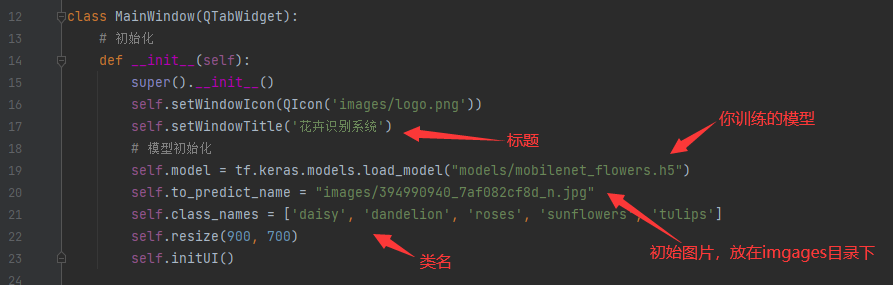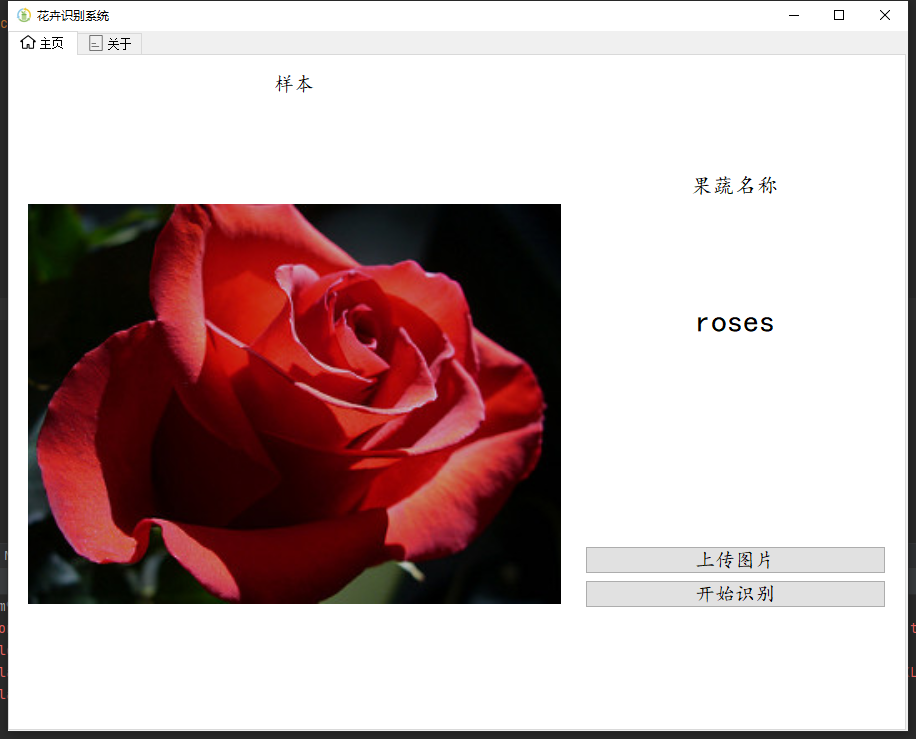# 手把手教你用tensorflow2.3训练自己的分类数据集

tensorflow2.x版本对小白非常友好，2.x的api中对keras进行了合并，大家只需要安装tensorflow就可以使用里面封装好的keras，利用keras可以快速地加载数据集和构建模型，下面我们直接来看以下通过tensorflow2.3训练自己的分类数据集吧。

vegetables_tf2.3: 基于tensorflow2.3开发的水果蔬菜识别系统 (gitee.com)

## 数据集收集

### 开源数据集

UCI Machine Learning Repository

CSDN – 专业开发者社区### 自行采集数据集

``````import requests
import re
import os

'User-Agent': 'Mozilla/5.0 (Windows NT 10.0; Win64; x64) AppleWebKit/537.36 (KHTML, like Gecko) Chrome/84.0.4147.125 Safari/537.36'}
name = input('请输入要爬取的图片类别：')
num = 0
num_1 = 0
num_2 = 0
x = input('请输入要爬取的图片数量？（1等于60张图片，2等于120张图片）：')
list_1 = []
for i in range(int(x)):
name_1 = os.getcwd()
name_2 = os.path.join(name_1, 'data/' + name)
url = 'https://image.baidu.com/search/flip?tn=baiduimage&ie=utf-8&word=' + name + '&pn=' + str(i * 30)
htlm_1 = res.content.decode()
a = re.findall('"objURL":"(.*?)",', htlm_1)
if not os.path.exists(name_2):
os.makedirs(name_2)
for b in a:
try:
b_1 = re.findall('https:(.*?)&', b)
b_2 = ''.join(b_1)
if b_2 not in list_1:
num = num + 1
img = requests.get(b)
f = open(os.path.join(name_1, 'data/' + name, name + str(num) + '.jpg'), 'ab')
print('---------正在下载第' + str(num) + '张图片----------')
f.write(img.content)
f.close()
list_1.append(b_2)
elif b_2 in list_1:
num_1 = num_1 + 1
continue
except Exception as e:
print('---------第' + str(num) + '张图片无法下载----------')
num_2 = num_2 + 1
continue

print('下载完成,总共下载{}张,成功下载:{}张,重复下载:{}张,下载失败:{}张'.format(num + num_1 + num_2, num, num_1, num_2))
``````

## 数据集整理

### 放置到相应的子文件夹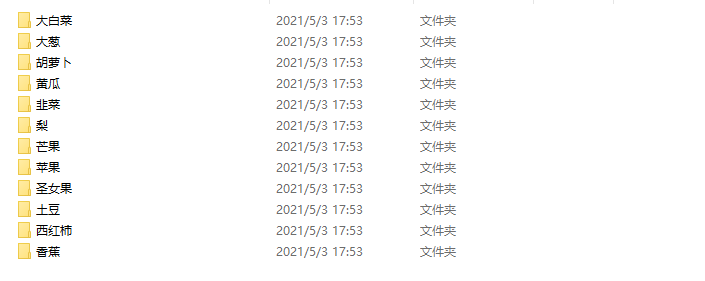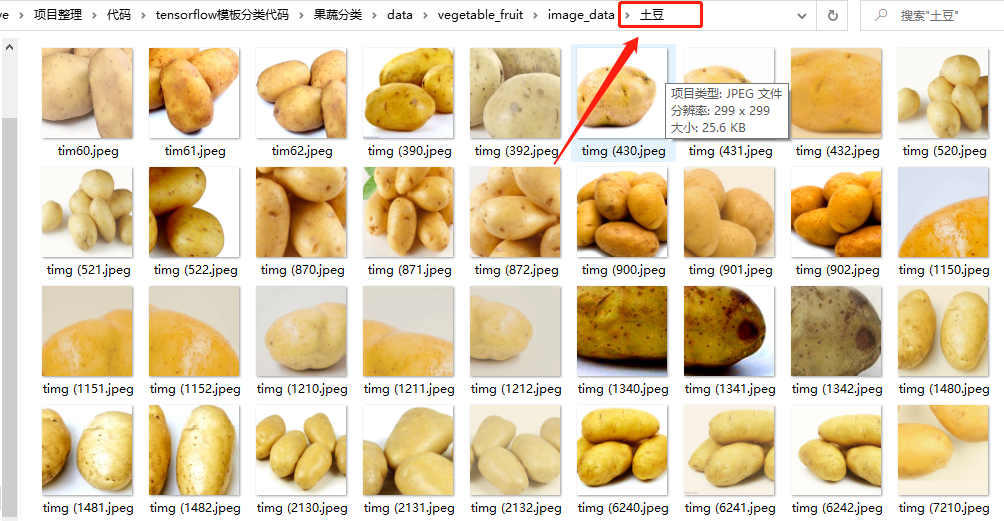### 划分训练集和测试集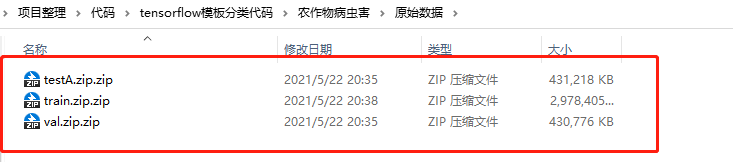``````# 作者: 宋老狗
import os
import random
from shutil import copy2

def data_set_split(src_data_folder, target_data_folder, train_scale=0.8, val_scale=0.0, test_scale=0.2):
'''
读取源数据文件夹，生成划分好的文件夹，分为trian、val、test三个文件夹进行
:param src_data_folder: 源文件夹 E:/biye/gogogo/note_book/torch_note/data/utils_test/data_split/src_data
:param target_data_folder: 目标文件夹 E:/biye/gogogo/note_book/torch_note/data/utils_test/data_split/target_data
:param train_scale: 训练集比例
:param val_scale: 验证集比例
:param test_scale: 测试集比例
:return:
'''
print("开始数据集划分")
class_names = os.listdir(src_data_folder)
# 在目标目录下创建文件夹
split_names = ['train', 'val', 'test']
for split_name in split_names:
split_path = os.path.join(target_data_folder, split_name)
if os.path.isdir(split_path):
pass
else:
os.mkdir(split_path)
# 然后在split_path的目录下创建类别文件夹
for class_name in class_names:
class_split_path = os.path.join(split_path, class_name)
if os.path.isdir(class_split_path):
pass
else:
os.mkdir(class_split_path)

# 按照比例划分数据集，并进行数据图片的复制
# 首先进行分类遍历
for class_name in class_names:
current_class_data_path = os.path.join(src_data_folder, class_name)
current_all_data = os.listdir(current_class_data_path)
current_data_length = len(current_all_data)
current_data_index_list = list(range(current_data_length))
random.shuffle(current_data_index_list)

train_folder = os.path.join(os.path.join(target_data_folder, 'train'), class_name)
val_folder = os.path.join(os.path.join(target_data_folder, 'val'), class_name)
test_folder = os.path.join(os.path.join(target_data_folder, 'test'), class_name)
train_stop_flag = current_data_length * train_scale
val_stop_flag = current_data_length * (train_scale + val_scale)
current_idx = 0
train_num = 0
val_num = 0
test_num = 0
for i in current_data_index_list:
src_img_path = os.path.join(current_class_data_path, current_all_data[i])
if current_idx <= train_stop_flag:
copy2(src_img_path, train_folder)
# print("{}复制到了{}".format(src_img_path, train_folder))
train_num = train_num + 1
elif (current_idx > train_stop_flag) and (current_idx <= val_stop_flag):
copy2(src_img_path, val_folder)
# print("{}复制到了{}".format(src_img_path, val_folder))
val_num = val_num + 1
else:
copy2(src_img_path, test_folder)
# print("{}复制到了{}".format(src_img_path, test_folder))
test_num = test_num + 1

current_idx = current_idx + 1

print("*********************************{}*************************************".format(class_name))
print(
"{}类按照{}：{}：{}的比例划分完成，一共{}张图片".format(class_name, train_scale, val_scale, test_scale, current_data_length))
print("训练集{}：{}张".format(train_folder, train_num))
print("验证集{}：{}张".format(val_folder, val_num))
print("测试集{}：{}张".format(test_folder, test_num))

if __name__ == '__main__':
src_data_folder = "C:/Users/Scm97/Desktop/dejahu/data"  # todo 原始数据集目录
target_data_folder = "C:/Users/Scm97/Desktop/dejahu/split_data"  # todo 数据集分割之后存放的目录
data_set_split(src_data_folder, target_data_folder)

``````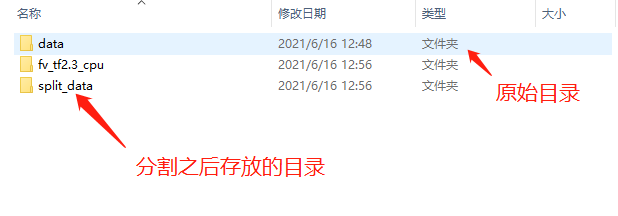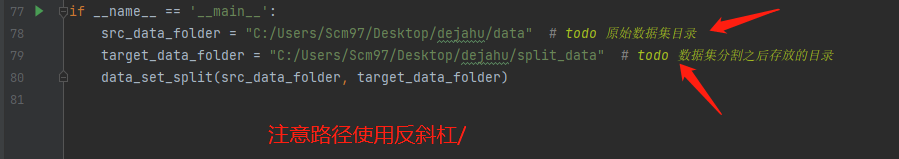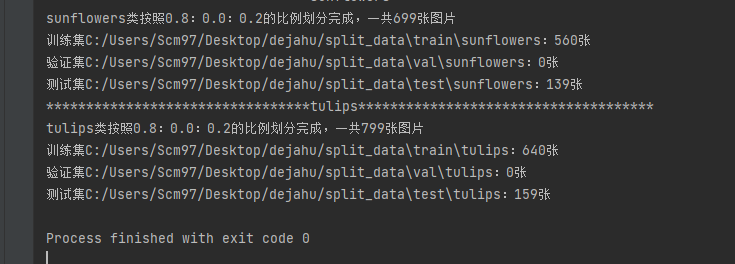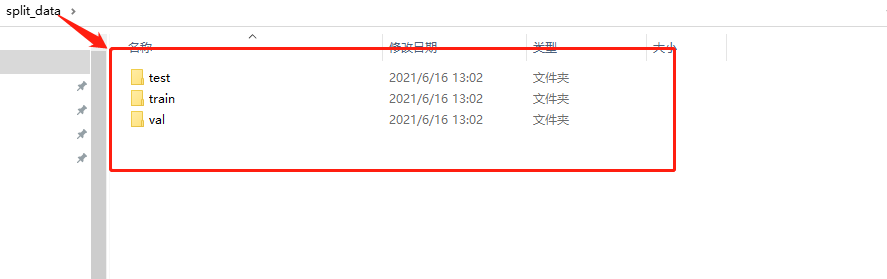## 训练模型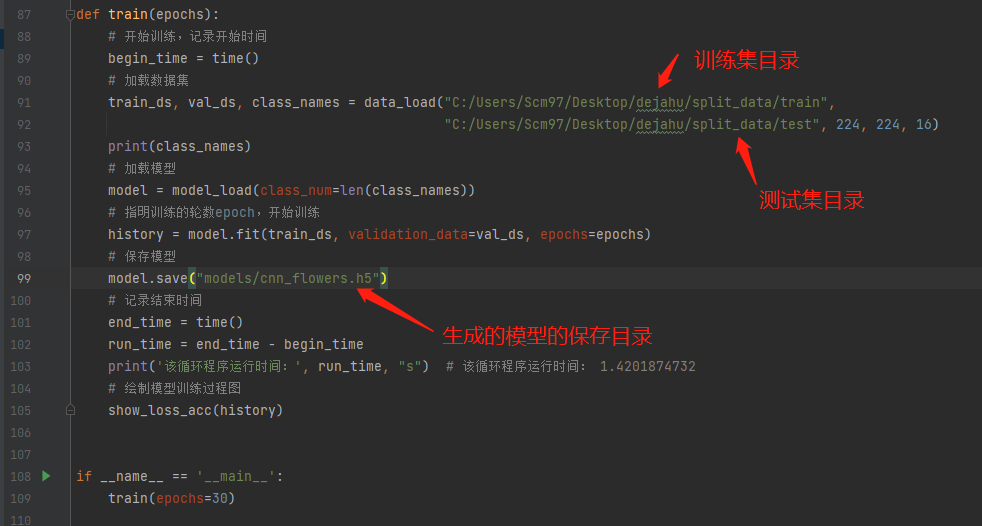`train_mobilnet.py`是训练mobilenet模型的代码，训练的模型将会保存在models目录下，这里也是只需修改三处即可。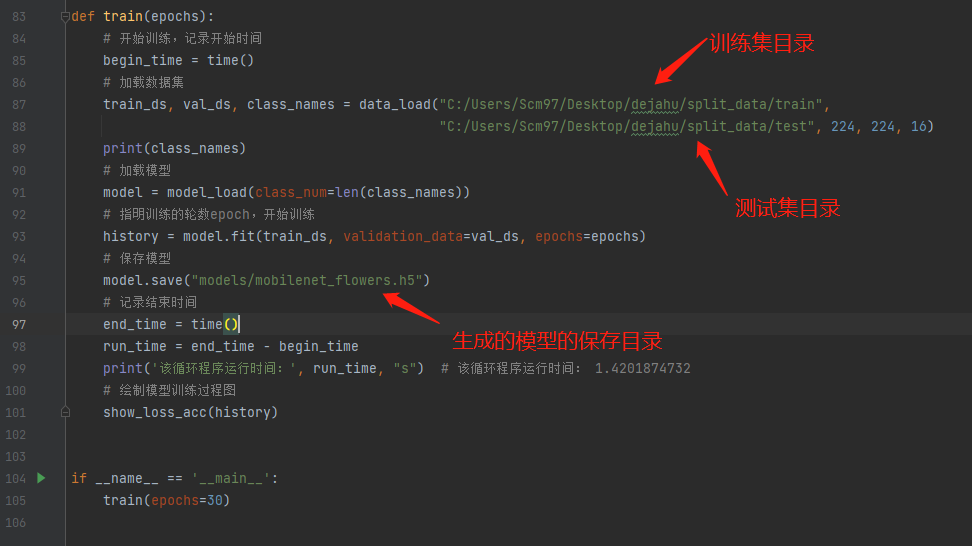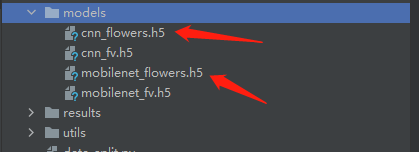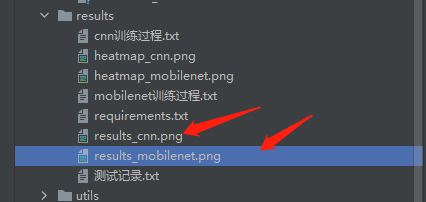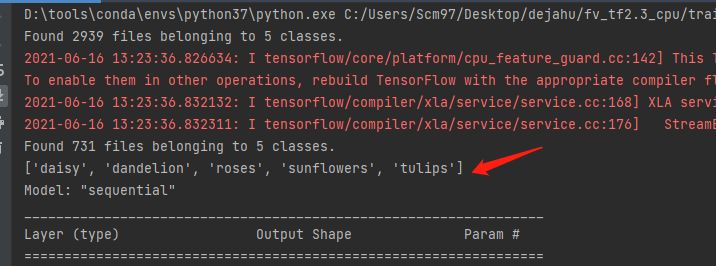## 测试模型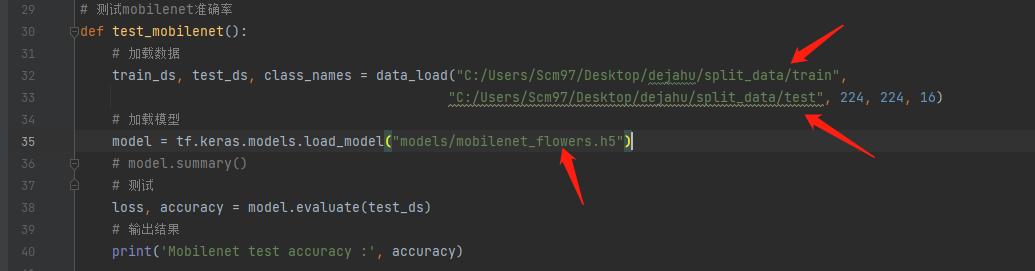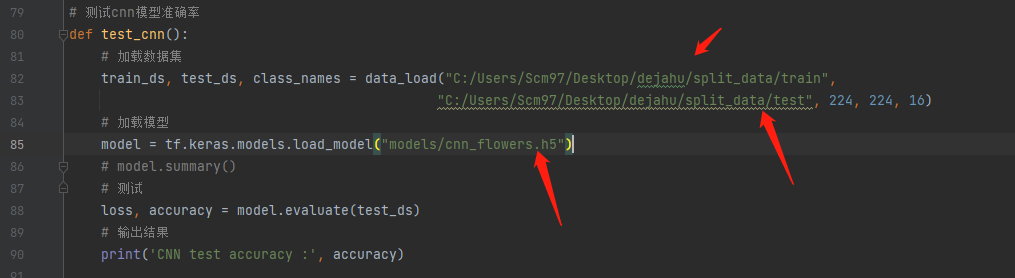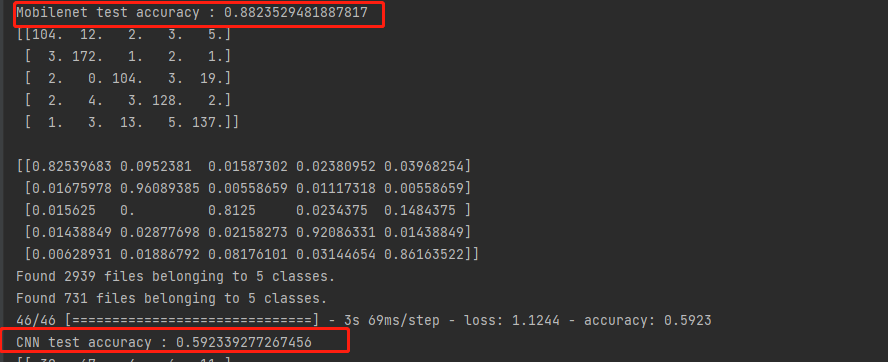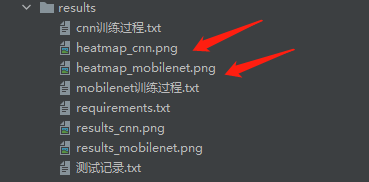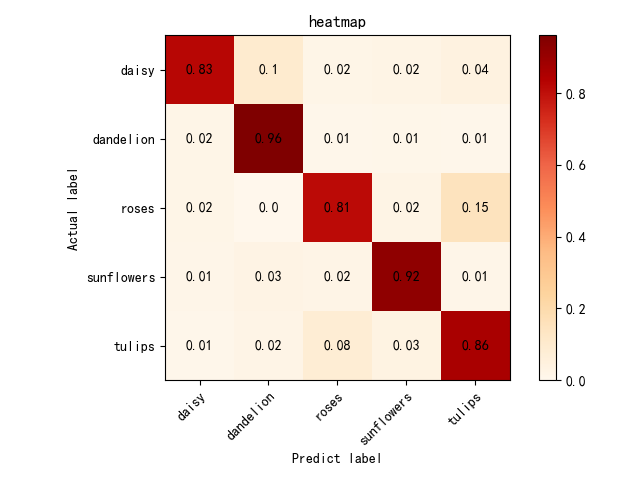## 使用模型

`window.py`代码中修改四处即可完成基本功能